## == and ===

### ==

The == operator will compare for equality after doing any necessary type conversions.

### ===

The === operator will not do the conversion, so if two values are not the same type === will simply return false

## compare

``````'' == '0'           // false
0 == ''             // true
0 == '0'            // true

false == 'false'    // false
false == '0'        // true

false == undefined  // false
false == null       // false
null == undefined   // true

' \t\r\n ' == 0     // true
``````

``````if(testObject){
// do something if testObject != null
}
``````

### NaN

``````NaN == NaN;        // false
``````

### boolean

``````2 == true;        // 2 === 1, false
2 == false;        // 2 === 0, false

1 == true;        // 1 === 1, true
0 == false;        // 0 === 0, true

'' == false;    // 0 === 0, true
'1' == true ;    // 1 === 1, true
'0' == false;    // 0 === 0, true
'test' == true    // NaN === 1, false
``````

### object

``````{} =='[object Objec]';    // true
['123'] == 123;            // true
[] == 0;                // true
``````

## JS學習推薦

• JavaScript設計模式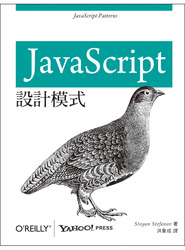• JavaScript優良部分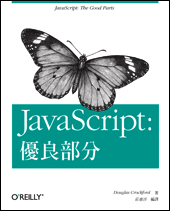• JavaScript深入精要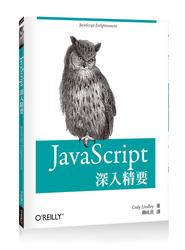• Speaking JavaScript｜簡明完整的 JS 精要指南 (Speaking JavaScript)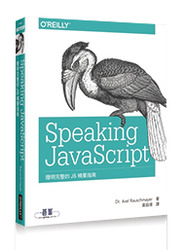• JavaScript高性能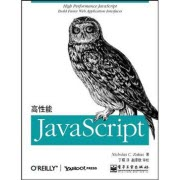• Object-Oriented JavaScript - Second Edition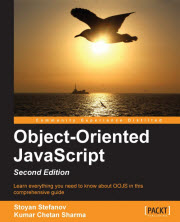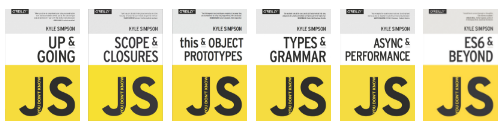P.S. 大師包含國外與國內這些願意寫書分喜的作家們